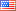Question-and-Answer Resource for the Building Energy Modeling Community
Get started with the Help page

Revision history [back]

PMV-PPD Mistake in Energyplus

When I read the Pmv-Ppd values in the Energyplus program, I get values that do not fit the Fanger equation. For example, PMV is -0.3, PPD is 33% or PMV is -0.5, PPD is 38%. Do you think these results are correct?

PMV-PPD Mistake in Energyplus

When I read the Pmv-Ppd values in the Energyplus program, I get values that do not fit the Fanger equation. For example, PMV is -0.3, PPD is 33% or PMV is -0.5, PPD is 38%. Do you think these results are correct?

PMV-PPD Mistake in Energyplus

When I read the Pmv-Ppd values in the Energyplus program, I get values that do not fit the Fanger equation. For example, PMV is -0.3, PPD is 33% or PMV is -0.5, PPD is 38%. Do you think these results are correct?

PMV-PPD Mistake in Energyplus

When I read the Pmv-Ppd values in the Energyplus program, I get values that do not fit the Fanger equation. For example, PMV is -0.3, PPD is 33% or PMV is -0.5, PPD is 38%. Do you think these results are correct?

PMV-PPD Mistake in Energyplus

When I read the Pmv-Ppd values in the Energyplus program, I get values that do not fit the Fanger equation. For example, PMV is -0.3, PPD is 33% or PMV is -0.5, PPD is 38%. Do you think these results are correct?6 retagged__AmirRoth__4388 ●5 ●16 http://bleedinggreenna...

PMV-PPD Mistake in Energyplus

When I read the Pmv-Ppd values in the Energyplus program, I get values that do not fit the Fanger equation. For example, PMV is -0.3, PPD is 33% or PMV is -0.5, PPD is 38%. Do you think these results are correct?